扇面形散射元构建的二维光子晶体慢光模拟研究Simulation Study on Slow Light of 2-D Photonic Crystals by Sector Scatterers

• 全文下载: PDF(1908KB)    PP.386-397   DOI: 10.12677/APP.2018.88049
• 下载量: 289  浏览量: 440   国家自然科学基金支持

In this paper, the air hole photonic crystal model is constructed by sector scatterers, and the linear defect waveguide is constructed. The dispersion curves in TM mode are simulated by using plane wave expansion method, and the group refractive index and group velocity are obtained by changing the parameters of scattering elements. The ideal model with large group refractive index and large bandwidth is obtained by normalized delay bandwidth product and dispersion constant. The waveguide structure is changed as a whole, and the scattering elements are divided into three types: face to face type, back to back type and rotation 30˚. The calculated results are compared and analyzed.

1. 引言

2. 模型结构

3. 模拟分析

3.1. 慢光中的基本概念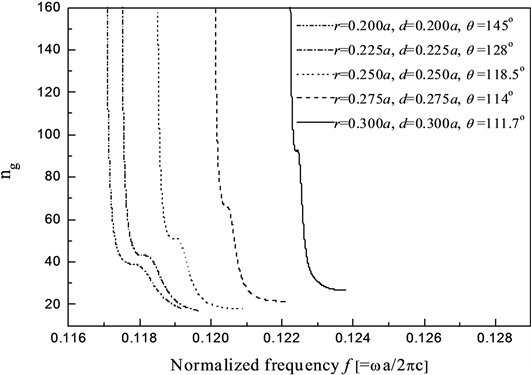Figure 1. Sector scatterer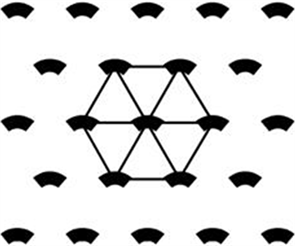Figure 2. Photonic crystal with hexagonal lattice structure

${n}_{g}=\frac{c}{{v}_{g}}=c\frac{\text{d}k}{\text{d}\omega }$ (1)

${n}_{g}={n}_{eff}+\omega \frac{\text{d}{n}_{eff}}{\text{d}\omega }$ (2)

${n}_{g}\approx \omega \frac{\Delta n}{\Delta \omega }$ (3)

${n}_{g}\frac{\Delta \omega }{\omega }\approx \Delta n$ (4)

$\Delta {n}_{\mathrm{max}}=\frac{{k}_{\mathrm{max}}}{{f}_{1}}-\frac{{k}_{\mathrm{min}}}{{f}_{2}}\approx \frac{{k}_{\mathrm{max}}-{k}_{\mathrm{min}}}{{f}_{1}}$ (5)

$D=\frac{1}{c}\frac{\text{d}{n}_{g}}{\text{d}\lambda }$ (6)

3.2. 色散曲线的规律分析

3.3. 面对面型线缺陷波导的慢光特性分析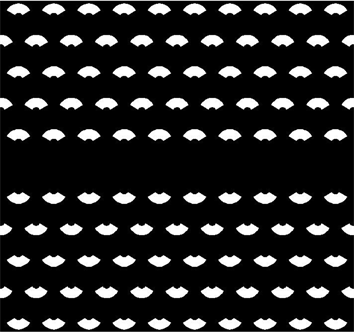Figure 3. Face-to-face line defect waveguide structure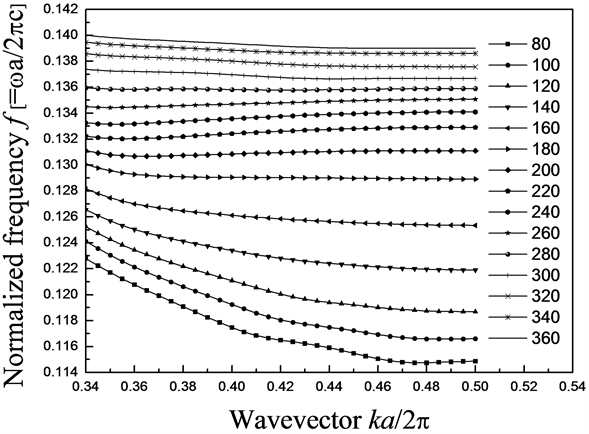Figure 4. Relationship of dispersion curve with θ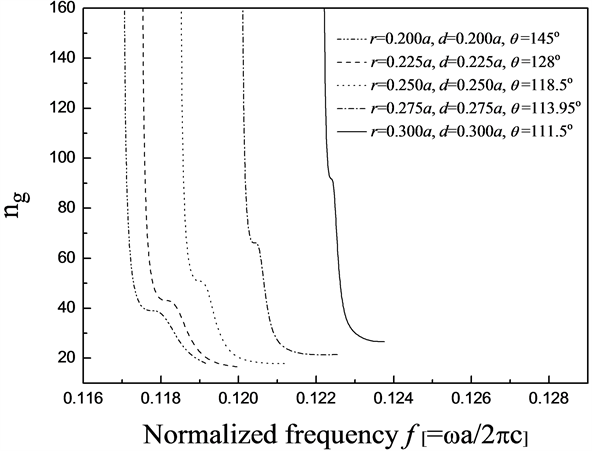Figure 5. Group refractive index curve of face to face line defect waveguidesTable 1. n g , Δ λ , n g Δ ω / ω , v g in face-to-face line defect waveguide

D的单位是ps/mm∙nm，计算当 $|D|\le 1$ 时，波长的变化范围，即带宽，如表2所示，其最大值可达3.1 nm。若令 $f=1\text{\hspace{0.17em}}\text{Thz}$ ，带宽可达1641.2 nm。

3.4. 背对背型线缺陷波导的慢光特性分析

$r=0.3a,d=0.3a,\theta ={111.7}^{\circ }$ 时， ${n}_{g}=92.6$ ，为最大值。群折射率可以实现39.0~92.6的可调范围。Table 2. a and Δ λ for | D | ≤ 1 in face to face line defect waveguides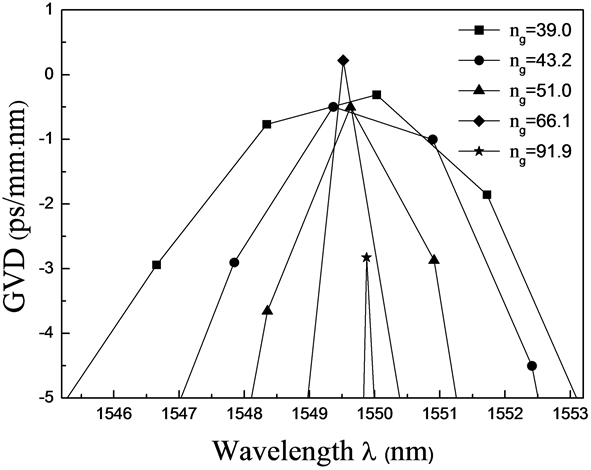Figure 6. GVD curves of face to face line defect waveguides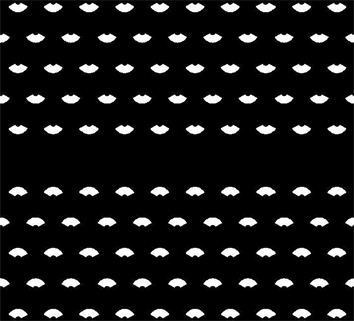Figure 7. Back-to-back line defect waveguide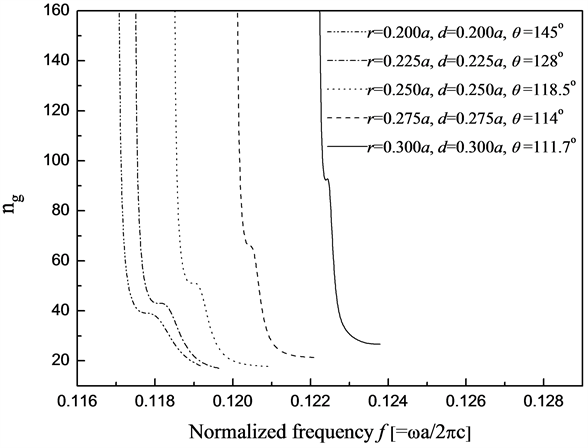Figure 8. Group refractive index curve of back-to-back linear defect waveguidesTable 3. n g , Δ λ , n g Δ ω / ω , v g in back-to-back face line defect waveguides

$\lambda =1550\text{\hspace{0.17em}}\text{nm}$$\Delta {n}_{g}=±10%$ ，计算其色散常数D，如图9所示。由表中数据得， $|D|\le 1$ 时，最大带宽可达2.73 nm。取 $f=1\text{\hspace{0.17em}}\text{Thz}$ ，最大带宽可达1649.6 nm。

3.5. 旋转30˚后线缺陷波导的慢光特性分析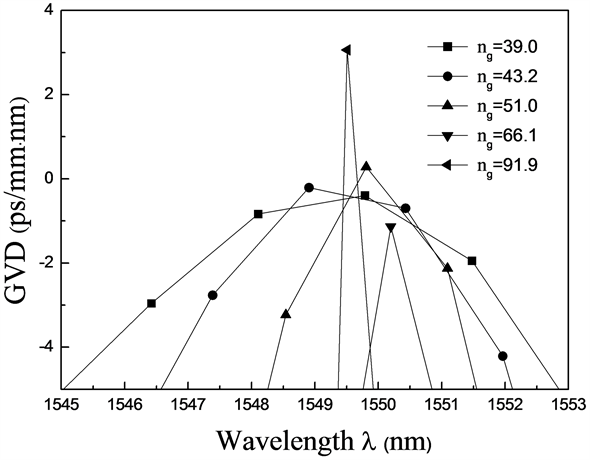Figure 9. GVD curve of a back-to-back line defect waveguideTable 4. a and Δ λ for | D | ≤ 1 in back-to-back line defect waveguidesFigure 10. Rotating 30˚ post-line defective waveguide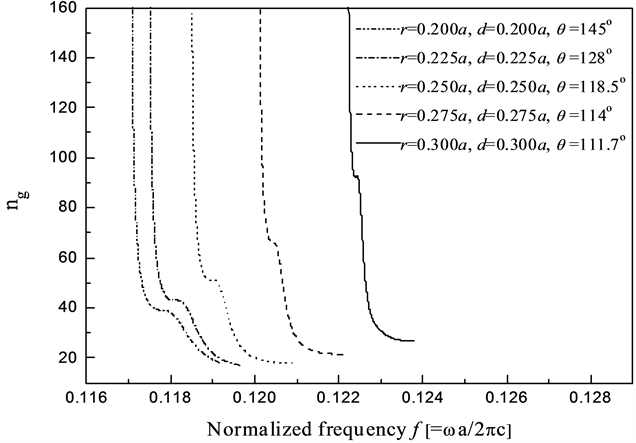Figure 11. The group refractive index curve of a linear defect waveguide after rotation 30˚Table 5. n g , Δ λ , n g Δ ω / ω , v g in a linear defect waveguide after rotation 30˚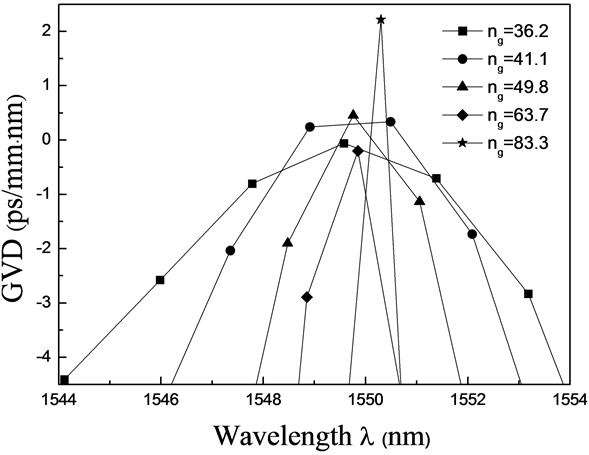Figure 12. GVD curve of the waveguide with line defects after rotation 30˚Table 6. a and Δ λ in a linear defect waveguide after rotation 30˚ when | D | ≤ 1

3.6. 三种模型的比较分析

4. 结论

  李正华, 薛燕陵, 沈廷根. 光子晶体内的慢光及其应用[J]. 激光与红外, 2008, 38(1): 3-6.  陈培专, 侯国付, 索松, 等. 基于一维光子晶体的新型背反射器及其在非晶硅薄膜太阳电池中的应用[J]. 物理学报, 2014, 63(7): 077301.  朱志宏, 叶卫民, 袁晓东, 等. 光子晶体波导定向耦合器[J]. 光学学报, 2003, 23(10): 1237-1240.  Yablonovitch, E. (1987) Inhibited Spontaneous Emission in Solid-State Physics and Electronics. Physical Review Letters, 58, 2059-2062. https://doi.org/10.1103/PhysRevLett.58.2059  John, S. (1987) Strong Localization of Photons in Certain Disordered Die-lectric Superlattices. Physical Review Letters, 58, 2486-2489. https://doi.org/10.1103/PhysRevLett.58.2486  刘会, 刘丹, 赵恒, 等. 空气环型二维光子晶体完全带隙特性研究[J]. 物理学报, 2013, 62(19): 194208.  邓开发, 是度芳, 蒋美萍, 等. 光子晶体研究进展[J]. 量子电子学报, 2004, 21(5): 555-564.  蔡青, 黄昌清, 梁培, 等. 基于平面波展开法的二维光子晶体表面模式研究[J]. 光子学报, 2012, 41(4): 430-435.  陈海燕, 黄小莉, 刘永智. 平面光波导光场分布的有限时域差分法计算[J]. 光子学报, 2001, 30(2): 214-217.  张小贝, 黄德修, 洪伟, 等. 传输矩阵法分析微环谐振器阵列传输特性[J]. 光学学报, 2007, 27(9): 1585-1592.  Chassagneux, Y., Colombeli, R., Maineult, W., et al. (2009) Electrically Pumped Photonic Crystal Terahertz Lasers Controlled by Boundary Condition. Nature, 457, 174-178. https://doi.org/10.1038/nature07636  刘建静, 郝伟, 刘锴, 等. 光折变材料的带隙结构研究[J]. 量子光学学报, 2007, 13(1): 47-51.  Liang, Q., Li, D. and Yang, G. (2012) Fabrication of Diamond-Structured Multiceramic Coupling Photonic Crystal and Its Ultra-Wide Bandgap Properties. Microwave and Optical Technology Letters, 54, 2569-2572. https://doi.org/10.1002/mop.27134  Wan, Y., Fu, K., Li, C. and Yun, M. (2013) Improving Slow Light Effect in Photonic Crystal Line Defect Waveguide by Using Eye-Shaped Scatterers. Optics Communications, 286, 192-196. https://doi.org/10.1016/j.optcom.2012.09.025  万勇, 付凯, 云茂金, 郭月, 夏临华. 调整圆弓形散射元参数实现低群速和低色散的慢光效应[J]. 中国激光, 2013, 40(10): 1-7.  万勇, 韩文娟, 贾明辉, 云茂金, 郭月, 孙蕾. 圆弓形散射元构建的耦合波导的慢光特性研究[J]. 光学学报, 2015, 35(3): 0313001.  Wan, Y., Ge, X., Xu, S., Guo, Y. and Yuan, F. (2017) Ul-tra-Slow Light Effects in Symmetric and Asymmetric Waveguide Structures with Moon-Like Scatters. Frontier of Physics, 12, Article ID: 124204.  Wan, Y., Xu, S., Li, M.-X., Liu, M.-N., Jiang, C.-Y. and Yuan, F. (2018) Slow Light Effect with High Group Index and Wideband by Saddle-Like Mode in PC-CROW. Frontier of Physics, 13, Article ID: 134202.  Wang, D., Zhang, J., Yuan, L., et al. (2011) Slow Light Engineering in Polyatomic Photonic Crystal Waveguides Based on Square Lattice. Optics Com-munications, 284, 5829-5832. https://doi.org/10.1016/j.optcom.2011.07.080  池灏, 蒋铭, 赵焕东, 等. 光子晶体光纤的非线性效应及其应用研究进展[J]. 半导体光电, 2003, 24(5): 297-300.  吴冰冰, 何晓东, 薛伟, 等. 一位非线性光子晶体全光开关的数值研究[J]. 光子学报, 2009, 38(2): 293-297.  Wan, Y., Ge, S., Guo, Y., et al. (2014) Application of 2D Graded Eye-Shape Scatters for Slow Light Effect in Photonic Crystal Line Defect Waveguide. Optik, 125, 1605-1609. https://doi.org/10.1016/j.ijleo.2013.10.015  Li, M., Li, L., Zhang, X., et al. (2013) The Slow Light in the Closed-Packed Face-Centered Cubic Photonic Crystal: Characteristics and Application Design. Optical and Quantum Electronics, 45, 1107-1113. https://doi.org/10.1007/s11082-013-9727-8